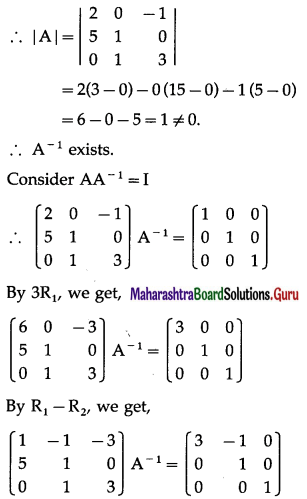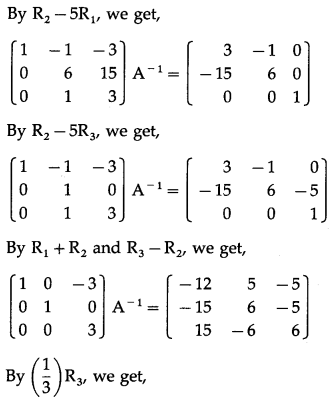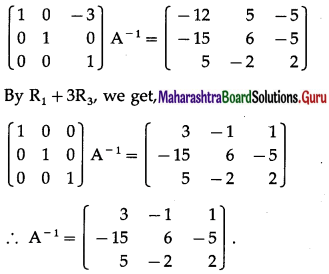# Maharashtra Board 12th Maths Solutions Chapter 2 Matrices Ex 2.2

Balbharti 12th Maharashtra State Board Maths Solutions Book Pdf Chapter 2 Matrices Ex 2.2 Questions and Answers.

## Maharashtra State Board 12th Maths Solutions Chapter 2 Matrices Ex 2.2

Question 1.
Find the co-factors of the elements of the following matrices
(i) $$\left[\begin{array}{cc} -1 & 2 \\ -3 & 4 \end{array}\right]$$
Solution:
Let A = $$\left[\begin{array}{cc} -1 & 2 \\ -3 & 4 \end{array}\right]$$
Here, a11 = -11, M11 = 4
∴ A11 = (-1)1+1(4) = 4
a12 = 2, M12 = -3
∴ A12 = (-1)1+2(- 3) = 3
a21 = – 3, M21 = -2
∴ A21 = (- 1)2+1(2) = -2
a22 = 4, M22 = -1
∴ A22 = (-1)2+2(-1) = -1.

(ii) $$\left[\begin{array}{ccc} 1 & -1 & 2 \\ -2 & 3 & 5 \\ -2 & 0 & -1 \end{array}\right]$$
Solution:
Let A = $$\left[\begin{array}{ccc} 1 & -1 & 2 \\ -2 & 3 & 5 \\ -2 & 0 & -1 \end{array}\right]$$
The co-factor of aij is given by Aij = (-1)i+jMijQuestion 2.
Find the matrix of co-factors for the following matrices
(i) $$\left[\begin{array}{rr} 1 & 3 \\ 4 & -1 \end{array}\right]$$
Solution:
Let A = $$\left[\begin{array}{rr} 1 & 3 \\ 4 & -1 \end{array}\right]$$
Here, a11 = 1, M11 = -1
∴ A11 = (-1)1+1(-1) = -1
a12 = 3, M12 = 4
∴ A12 = (-1)1+2(4) = -4
a21 = 4, M21 = 3
∴ A21 = (-1)2+1(3) = -3
a22 = -1, M22 = 1
∴ A22 = (-1)2+1(1) = 1
∴ the co-factor matrix = $$\left[\begin{array}{ll} A_{11} & A_{12} \\ A_{21} & A_{22} \end{array}\right]$$
= $$\left(\begin{array}{rr} -1 & -4 \\ -3 & 1 \end{array}\right)$$

(ii) $$\left[\begin{array}{rrr} 1 & 0 & 2 \\ -2 & 1 & 3 \\ 0 & 3 & -5 \end{array}\right]$$
Solution:
Let A = $$\left[\begin{array}{rrr} 1 & 0 & 2 \\ -2 & 1 & 3 \\ 0 & 3 & -5 \end{array}\right]$$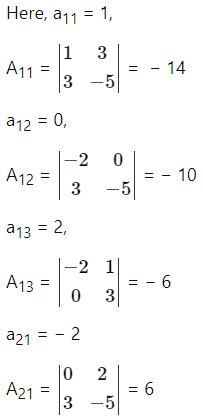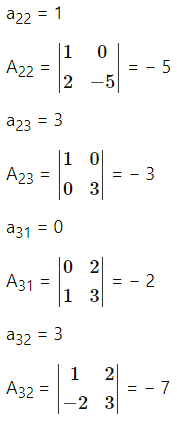A11 = -14, A12 = -10, A13 = -6,
A21 = 6, A22 = -5, A23 = -3,
A31 = -2, A32 = -7, A33 = 1.
∴ the co-factor matrix
= $$\left[\begin{array}{lll} A_{11} & A_{12} & A_{13} \\ A_{21} & A_{22} & A_{23} \\ A_{31} & A_{32} & A_{33} \end{array}\right]$$ = $$\left[\begin{array}{rrr} -14 & -10 & -6 \\ 6 & -5 & -3 \\ -2 & -7 & 1 \end{array}\right]$$Question 3.
Find the adjoint of the following matrices.
(i) $$\left[\begin{array}{cc} 2 & -3 \\ 3 & 5 \end{array}\right]$$
Solution:
Let A = $$\left[\begin{array}{cc} 2 & -3 \\ 3 & 5 \end{array}\right]$$
Here, a11 = 2, M11= 5
∴ A11 = (-1)1+1(5) = 5
a12 = -3, M12 = 3
∴ A12 = (-1)1+2(3) = -3
a21 = 3, M21 = -3
∴ A A21 = (-1)2+1(-3) = 3
a22 = 5, M22 = 2
∴ A22 = (-1)2+1 = 2
∴ the co-factor matrix = $$\left[\begin{array}{ll} A_{11} & A_{12} \\ A_{21} & A_{22} \end{array}\right]$$
= $$\left[\begin{array}{rr} 5 & -3 \\ 3 & 2 \end{array}\right]$$
∴ adj A = $$\left(\begin{array}{rr} 5 & 3 \\ -3 & 2 \end{array}\right)$$(ii) $$\left[\begin{array}{ccc} 1 & -1 & 2 \\ -2 & 3 & 5 \\ -2 & 0 & -1 \end{array}\right]$$
Solution:A11 = -3, A12 = -12, A13 = 6,
A21 = -1, A22 = 3, A23 = 2,
A31 = -11, A32 = -9, A33 = 1
∴ the co-factor matrix = $$\left[\begin{array}{lll} \mathrm{A}_{11} & \mathrm{~A}_{12} & \mathrm{~A}_{15} \\ \mathrm{~A}_{21} & \mathrm{~A}_{22} & \mathrm{~A}_{23} \\ \mathrm{~A}_{31} & \mathrm{~A}_{32} & \mathrm{~A}_{33} \end{array}\right]$$
= $$\left[\begin{array}{rrr} -3 & -12 & 6 \\ -1 & 3 & 2 \\ -11 & -9 & 1 \end{array}\right]$$
∴ adj A = $$\left[\begin{array}{rrr} -3 & -1 & -11 \\ -12 & 3 & -9 \\ 6 & 2 & 1 \end{array}\right]$$

Question 4.
If A = $$\left[\begin{array}{ccc} 1 & -1 & 2 \\ 3 & 0 & -2 \\ 1 & 0 & 3 \end{array}\right]$$, verify that A (adj A) = (adj A) A = | A | ∙ I
Solution:
A = $$\left[\begin{array}{ccc} 1 & -1 & 2 \\ 3 & 0 & -2 \\ 1 & 0 & 3 \end{array}\right]$$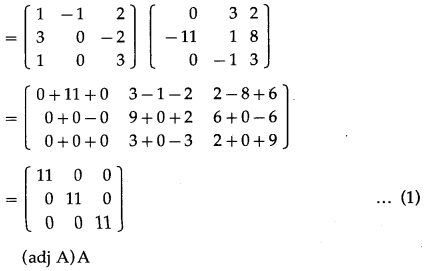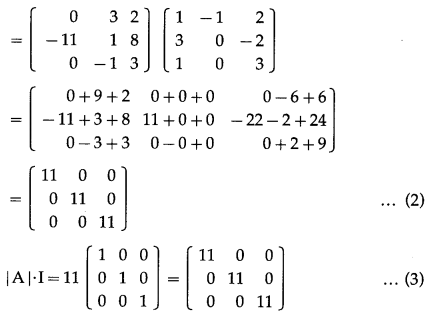From (1), (2) and (3), we get,
Note: This relation is valid for any non-singular matrix A.Question 5.
Find the inverse of the following matrices by the adjoint method
(i) $$\left[\begin{array}{ll} -1 & 5 \\ -3 & 2 \end{array}\right]$$
Solution:
Let A = $$\left[\begin{array}{ll} -1 & 5 \\ -3 & 2 \end{array}\right]$$
∴ |A| = $$\left|\begin{array}{ll} -1 & 5 \\ -3 & 2 \end{array}\right|$$ = -2 + 15 = 13 ≠ 0
∴ A-1 exists.
First we have to find the co-factor matrix
= [Aij]2×2, where Aij = (-1)i+jMij
Now, A11 = (-1)1+1M11 = 2
A12 = (-1)1+2M12 = -(-3) = 3
A21 = (-1)2+1M21 = -5
A22 = (-1)2+2M22 = -1
Hence, the co-factor matrix(ii) $$\left[\begin{array}{cc} 2 & -2 \\ 4 & 3 \end{array}\right]$$
Solution:
Let A = $$\left[\begin{array}{cc} 2 & -2 \\ 4 & 3 \end{array}\right]$$
|A| =  = 6 + 8 = 14 ≠ 0
∴ A-1 exist
First we have to find the co-factor matrix
= [Aij] 2×2 where Aij = (-1)i+jMij
Now, A11 = (-1)1+1M11 = 3
A12 = (-1)1+2M = -4
A21 = (-2)2+1M21 = (-2) = 2
A22 = (-1)2+2M22 = 2
Hence the co-factor matrix
= $$\left[\begin{array}{ll} A_{11} & A_{12} \\ A_{21} & A_{22} \end{array}\right]$$ = $$\left[\begin{array}{cc} 3 & -4 \\ 2 & 2 \end{array}\right]$$
∴ adj A = $$\left[\begin{array}{cc} 3 & 2 \\ -4 & 2 \end{array}\right]$$
∴ A-1 = $$\frac{1}{|\mathrm{~A}|}$$ (adj A) = $$\frac{1}{14}\left(\begin{array}{cc} 3 & 2 \\ -4 & 2 \end{array}\right)$$(iii) $$\left[\begin{array}{ccc} 1 & 0 & 0 \\ 3 & 3 & 0 \\ 5 & 2 & -1 \end{array}\right]$$
Solution:
Let A = $$\left[\begin{array}{ccc} 1 & 0 & 0 \\ 3 & 3 & 0 \\ 5 & 2 & -1 \end{array}\right]$$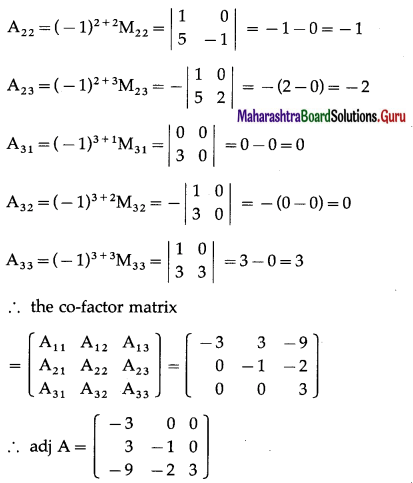∴ A-1 = $$\frac{1}{3}\left[\begin{array}{rrr} 3 & 0 & 0 \\ -3 & 1 & 0 \\ 9 & 2 & -3 \end{array}\right]$$

(iv) $$\left[\begin{array}{lll} 1 & 2 & 3 \\ 0 & 2 & 4 \\ 0 & 0 & 5 \end{array}\right]$$
Solution:
Let A = $$\left[\begin{array}{lll} 1 & 2 & 3 \\ 0 & 2 & 4 \\ 0 & 0 & 5 \end{array}\right]$$
∴ |A| = $$\left[\begin{array}{lll} 1 & 2 & 3 \\ 0 & 2 & 4 \\ 0 & 0 & 5 \end{array}\right]$$
= 1(10 – 0) – 0 + 0
= 1(10) – 0 + 0
= 10 ≠ 0
∴ A-1 exists.
First we have to find the co-factor matrix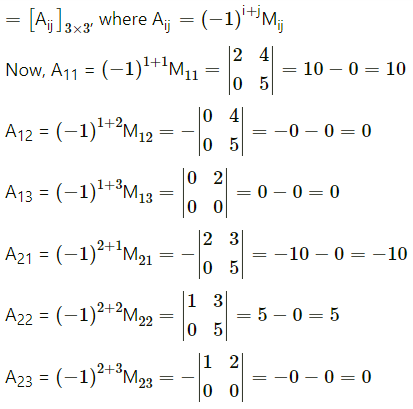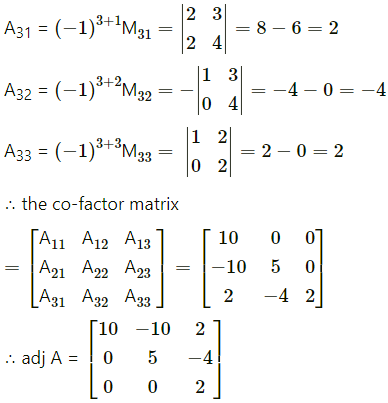∴ A-1 = $$\frac{1}{|\mathrm{~A}|}$$ (adj A)
= $$\frac{1}{10}\left(\begin{array}{rrr} 10 & -10 & 2 \\ 0 & 5 & -4 \\ 0 & 0 & 2 \end{array}\right)$$
∴ A-1 = $$\frac{1}{10}\left(\begin{array}{rrr} 10 & -10 & 2 \\ 0 & 5 & -4 \\ 0 & 0 & 2 \end{array}\right)$$Question 6.
Find the inverse of the following matrices
(i) $$\left[\begin{array}{cc} 1 & 2 \\ 2 & -1 \end{array}\right]$$
Solution:
Let A = $$\left[\begin{array}{cc} 1 & 2 \\ 2 & -1 \end{array}\right]$$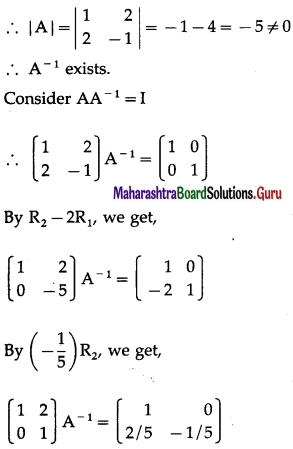(ii) $$\left[\begin{array}{cc} 2 & -3 \\ -1 & 2 \end{array}\right]$$
Solution:
Let A = $$\left[\begin{array}{cc} 2 & -3 \\ -1 & 2 \end{array}\right]$$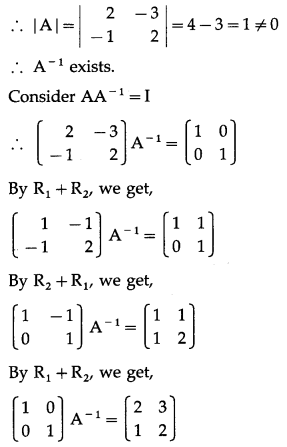∴ A-1 = $$\left(\begin{array}{ll} 2 & 3 \\ 1 & 2 \end{array}\right)$$(iii) $$\left[\begin{array}{lll} 0 & 1 & 2 \\ 1 & 2 & 3 \\ 3 & 1 & 1 \end{array}\right]$$
Solution:
Let A = $$\left[\begin{array}{lll} 0 & 1 & 2 \\ 1 & 2 & 3 \\ 3 & 1 & 1 \end{array}\right]$$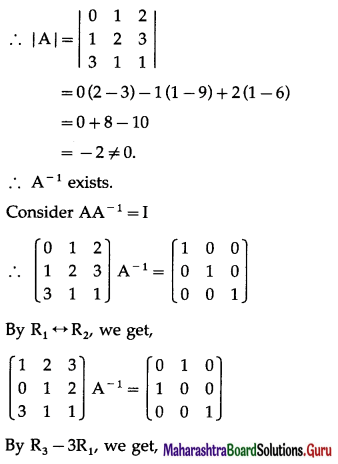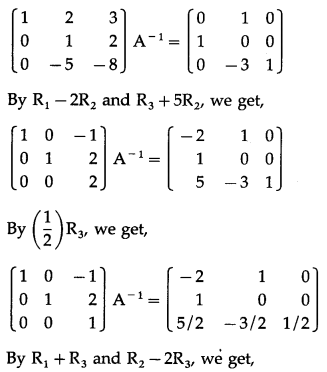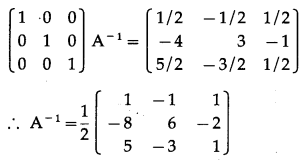(iv) $$\left[\begin{array}{ccc} 2 & 0 & -1 \\ 5 & 1 & 0 \\ 0 & 1 & 3 \end{array}\right]$$
Solution:
Let A = $$\left[\begin{array}{ccc} 2 & 0 & -1 \\ 5 & 1 & 0 \\ 0 & 1 & 3 \end{array}\right]$$